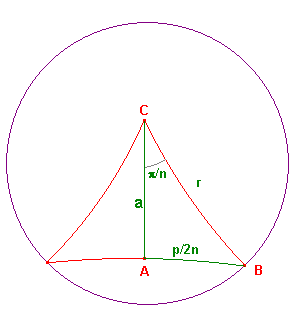# the circumference and area of hyperbolic circles

In euclidean geometry, the formulae for the circumference and area of a circle
can be obtained by considering the perimeter and area of a regular polygon
E(n) of n sides drawn within the circle. As n increases, the polygon expands
to fill the circle. Thus the limits as n -> ∞ give the circumference and area.

We use the same idea in hyperbolic geometry, though we replace E(n) by a
hyperbolic polygon H(n) symmetric about C, the hyperbolic centre of the circle.
If A and B are consecutive vertices of P(n), then <ACB is 2π/n.

 hyperbolic circle formulae A hyperbolic circle with hyperbolic radius r has hyperbolic length C(r) = 2πsinh(r), hyperbolic area D(r) = 4πsinh2(r/2). proof Suppose that the hyperbolic polygon H(n) introduced above has hyperbolic perimeter p and hyperbolic area d. The hyperbolic segments from the centre C to the vertices divide H(n) into n equal hyperbolic triangles. The figure on the right shows one traingle in red. the green segment is the perpendicular from C to the side of the polygon. By symmetry it bisects the side and the angle at C. Thus the hyperbolic triangle CAB has d(A,B) = p/2n, ∞. Clearly p -> C(r). By the limit lemma for sin and sinh, we get πsinh(r) = C(r)/2, which gives the required result for C(r). Since ΔABC is right-angled, the area formula gives sin(d/2n) = sinh(a)sinh(p/2n)/(cosh(r)+1), so that 2nsin(d/2n) = sinh(a)2nsinh(p/2n)/(cosh(r)+1). Now let n -> ∞. Then d -> D(r), a -> r and p -> C(r). By the limit lemma for sin and sinh, we get D(r) = sinh(r)C(r)/(cosh(r)+1) =2πsinh(r)sinh(r)/(cosh(r)+1). Now sinh(r) = 2sinh(r/2)cosh(r/2), and cosh(r)+1 = 2cosh2(r/2), so we get D(r) = 4πsinh2(r/2).hyperbolic area